# PC/CP320 Physical Computing

## Operational Amplifiers

### Objectives

An operational amplifier or op-amp is a high gain DC amplifier typically used in a closed-loop configuration. The objectives for this lab are:

• to investigate two common closed-loop op-amp circuits: the non-inverting amplifier and the voltage follower (buffer)

At completion of the lab, you should have a non-inverting amplifier circuit on a breadboard. Do not dismantle this circuit. Store this circuits in your lockup area for use later.  Since you are going to use this circuit in the coming weeks as a part of larger circuits, make sure you record all key connection points.

### Equipment

• bench supply, oscilloscope
• function generator
• for non-inverting amplifier: LM324: data sheet from local server

### Procedure

Since you will be having negative supply voltages that are not always the same as ground, use white wires for the negative supply voltage. There are white alligator clips to help with this.

1.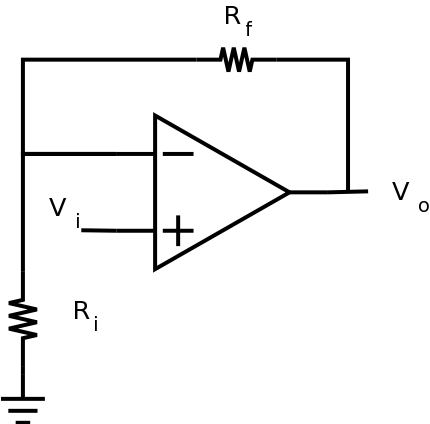The non-inverting amplifier circuit to the left produces an output signal, Vo, that is given by Vo = (Rf/ Ri + 1) Vin Since the input does not enter the inverting input, the output signal will be of the same polarity as the input signal.

If we would like the above circuit to have a gain of ten, what are the relationships between Ri and Rf?
What is the smallest gain which can be produced by this amplifier?
When Ri is infinite, and Rf equals zero, what circuit does this become?

2. Using the LM324, construct the non-inverting amplifier circuit shown above subject to the following constraints:
• gain is 10
• select resistors in the 1k to 100k range (record the actual resistor values for use in calculations)
• use 12V and GROUND for the supply voltages. (Single supply configuration).
• let Vin be a 100mV DC voltage.
Measure Vo.  Considering the input voltage, does the circuit work as you expect?  Compare the measured Vo with the calculated Vo

In order to show that the circuit works as expected, you'll need to display both the input and output at the same time.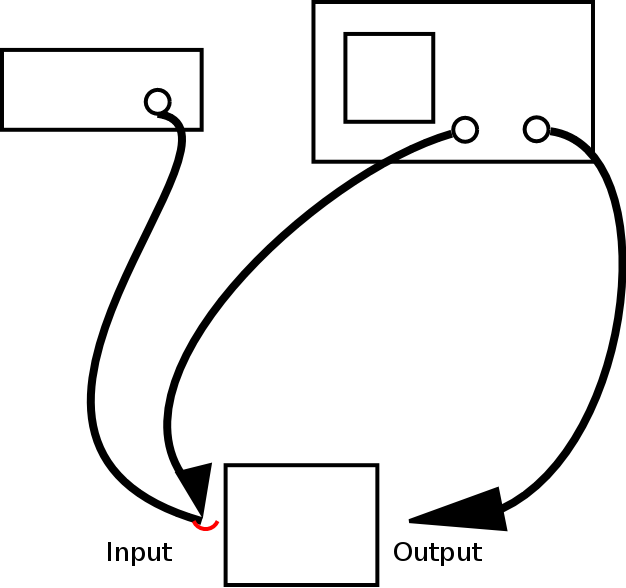Checkpoint: get lab supervisor to verify that you are getting the expected result before proceeding.

3. Change the circuit from part 2 to drive Vin with a 1kHz, 1Vp-p sine wave with a DC offset of 1V (verify signal is correct before applying to the circuit). What is the observed maximum output swing? Why did I choose a 1 Vp-p signal rather than a 5Vp-p signal?
Adjust the amplitude and/or DC offset of the input in order to answer the following: What are the limits of the input voltage which produce an undistorted output?

4. Change the circuit from part 3 to use supply voltages of +5V/0V.
As above, adjust the amplitude and/or DC offset of the input in order to answer the following: What are the limits of the input voltage which produce an undistorted output?
Is this consistent with the changes you made in the supply voltages?
The LM324 is known as a single supply op amp. Looking at your results, can you determine why it is designated that way? (Note: Any op amp can be used in either single supply or dual supply configuration, but there is a difference in how each will perform.)

5. Put a resistance substitution from the output of the op-amp to ground. Set the initial resistance to at least 1 kohm or so. Observe the output and see how it decreases as the resistance decreases. Find the value of resistance for which the output is half of what it was originally. Determine the current out of the op-amp for this resistance.
This gives a reasonable limit of the load which can be driven by the op-amp.

Demonstrate the non-inverting amplifier circuit to the lab supervisor.
In order to show that the circuit works as expected, you'll need to display both the input and output at the same time.

6. #### The voltage follower (buffer) - the simplest closed-loop configuration

The voltage follower (or buffer) is a very simple circuit where the output should "follow" the input. In other words, Vout should equal Vin. Is the voltage follower a special case of the non-inverting amplifier? Explain.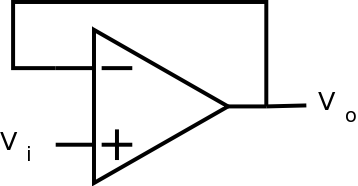Have the lab supervisor/demonstrator sign your lab notebook before you leave the lab.

## Resources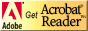If you need to update a browser, you might try Firefox which is
• free
• open source
• available for several platformsSince this page uses cascading style sheets for its layout, it will look best with a browser which supports the specifications as fully as possible.

If you are looking for an office package, with a word processor, spreadsheet, etc., you might try LibreOffice which is
• free
• open source
• available for several platforms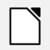Go to the main page for the Department of Physics and Computer Science.

Wilfrid Laurier University